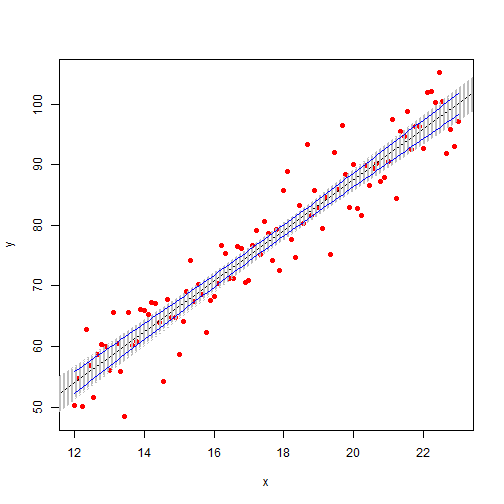# Simulating responses from a linear model

Say you fit a model using R's lm() function. What you get in return are coefficients that represent an estimate of the linear function that gave rise to the data. The assumption is the response of the model is normally distributed with a mean equal to the linear function and a standard deviation equal to the standard deviation of the residuals. Using notation we express this as follows for a simple linear model with intercept and slope coefficients:

$Y_{i} \sim N(\beta_{0} + \beta_{1}x_{i},\sigma)$

The implication of this in the world of statistical computing is that we can simulate responses given values of x. R makes this wonderfully easy with its simulate() function. Simply pass it the model object and the number of simulations you want and it returns a matrix of simulated responses. A quick example:

set.seed(1)
# generate data from the linear function y = 3 + 4.2x with random noise
x <- seq(12, 23, length = 100)
y <- 3 + 4.2 * x + rnorm(100, 0, 5)
mod <- lm(y ~ x)
summary(mod)

##
## Call:
## lm(formula = y ~ x)
##
## Residuals:
##     Min      1Q  Median      3Q     Max
## -11.700  -3.029   0.078   2.926  11.487
##
## Coefficients:
##             Estimate Std. Error t value Pr(>|t|)
## (Intercept)    3.900      2.504    1.56     0.12
## x              4.180      0.141   29.70   <2e-16 ***
## ---
## Signif. codes:  0 '***' 0.001 '**' 0.01 '*' 0.05 '.' 0.1 ' ' 1
##
## Residual standard error: 4.51 on 98 degrees of freedom
## Multiple R-squared:   0.9,   Adjusted R-squared:  0.899
## F-statistic:  882 on 1 and 98 DF,  p-value: <2e-16


Regressing y on x estimates the coefficients to be about 3.9 and 4.18, pretty close to the true values of 3 and 4.2. It also estimates the standard deviation of the error term to be 4.51, not too far from the 5 we specified in rnorm() when we generated the noise. Now let's use our model to simulate responses (i.e., y values).

head(simulate(mod, 5))

##   sim_1 sim_2 sim_3 sim_4 sim_5
## 1 51.26 55.90 58.09 58.91 54.40
## 2 54.71 62.14 49.79 63.08 53.18
## 3 50.87 62.15 63.88 52.26 49.64
## 4 56.16 53.96 53.72 53.69 55.50
## 5 52.96 45.60 63.38 54.04 60.39
## 6 64.35 67.65 63.20 54.68 63.57


The simulate() function returns a data frame. In this case the data frame has 100 rows and 5 columns. (I use the head() function to only show the first six rows.) Each column represents a simulated set of responses from our linear model given our x values. In the first row we have 5 values drawn from a Normal distribution with mean $$3.89 + 4.17x_{1}$$ and a standard deviation of 4.51. In the second row we have a value drawn from a Normal distribution with mean $$3.89 + 4.17x_{2}$$ and a standard deviation of 4.51. And so on. We could do this “manually” as follows:

simResp <- matrix(0, 100, 5)
for (i in 1:5) {
simResp[, i] <- rnorm(5, c(coef(mod) + coef(mod) * x), rep(summary(mod)$sigma, 5)) } simResp[1:6, ]  ## [,1] [,2] [,3] [,4] [,5] ## [1,] 52.52 48.93 54.80 52.14 52.90 ## [2,] 61.30 47.77 56.02 53.17 46.46 ## [3,] 57.37 53.98 53.25 46.90 63.04 ## [4,] 57.90 64.48 49.14 54.33 63.41 ## [5,] 55.30 56.91 67.99 54.80 59.03 ## [6,] 52.52 48.93 54.80 52.14 52.90  But the simulate() function is much faster and easier to use. Now 5 simulations is rather puny. A better number would be 1000. Then we can use our simulated y values to generate 1000 linear models, and thus have 1000 coefficient estimates. We can then use those estimates to create kernel density plots that allow us to visualize the amount of uncertainty in our coefficient estimates. Let's do that. Notice how I convert the output of the simulate() function to a matrix. That allows me to feed the 1000 responses to the lm() function in one fell swoop instead of coding a for loop to iterate through each column. simResp <- data.matrix(simulate(mod, 1000)) modSim <- lm(simResp ~ x)  modSim is the usual linear model object, but with the results of 1000 models instead of 1. Here are the coefficients for the first 5 models: coef(modSim)[1:2, 1:5]  ## sim_1 sim_2 sim_3 sim_4 sim_5 ## (Intercept) -0.5932 3.949 3.861 5.683 5.038 ## x 4.3836 4.166 4.199 4.062 4.113  Let's create a scatterplot of these coefficients. plot(modSim$coefficients[1, ], modSim$coefficients[2, ], xlab = "intercept", ylab = "slope", main = "Scatterplot of coefficients from simulated models")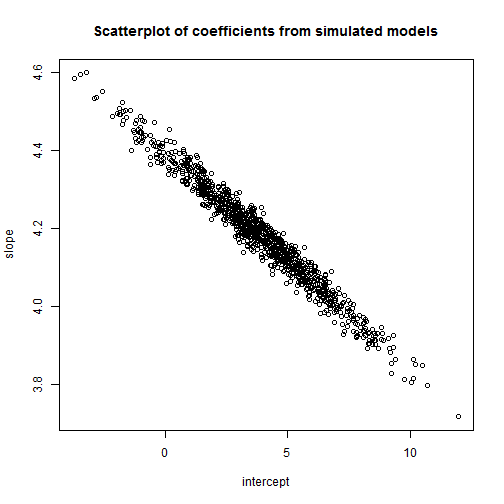We see that the variability of the slope estimates is pretty small (3.8 – 4.6), while the intercept estimates vary widely (from below 0 to 10). This jives with our original model (see output above) where the slope coefficient was signficant with a small standard error while the intercept was not significant and had a relatively large standard error. Next we create kernel density plots of our coefficient estimates. In addition I add a vertical line to represent the mean of the 1000 coefficient estimates and add the mean itself to the plot using the text() function. I use the difference in the range of the axes to allow me to automatically determine suitable coordinates. d1 <- density(modSim$coefficients[2, ])
d2 <- density(modSim$coefficients[1, ]) # slope plot(d1, main = "distribution of slope coefficients") abline(v = mean(modSim$coefficients[2, ]))
text(x = mean(modSim$coefficients[2, ]) + diff(range(d1$x)) * 0.05, y = diff(range(d1$y)) * 0.05, labels = round(mean(modSim$coefficients[2, ]), 2))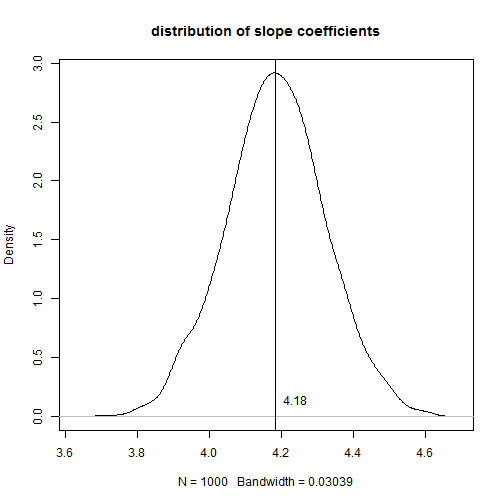# intercept
plot(d2, main = "distribution of intercept coefficients")
abline(v = mean(modSim$coefficients[1, ])) text(x = mean(modSim$coefficients[1, ]) + diff(range(d2$x)) * 0.05, y = diff(range(d2$y)) *
0.05, labels = round(mean(modSim$coefficients[1, ]), 2))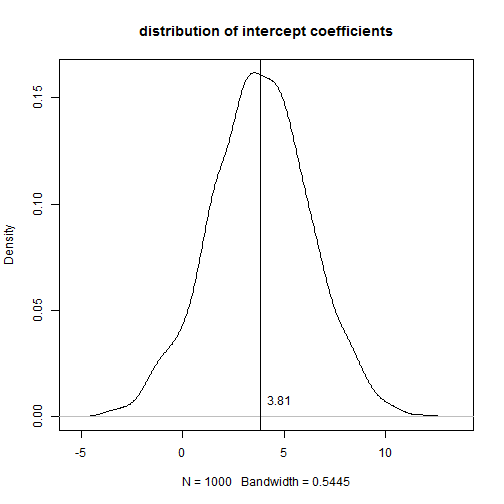If you don't compare the scale of the x-axis in the two plots the coefficients appear to have similar variability. Perhaps a better way to do it is to put both plots in one window and set the limits to the x-axis for both plots to be the range of the estimated intercept coefficients. par(mfrow = c(2, 1)) # slope plot(d1, main = "distribution of slope coefficients", xlim = range(d2$x))
abline(v = mean(modSim$coefficients[2, ])) text(x = mean(modSim$coefficients[2, ]) + diff(range(d2$x)) * 0.05, y = diff(range(d1$y)) *
0.05, labels = round(mean(modSim$coefficients[2, ]), 2)) # intercept plot(d2, main = "distribution of intercept coefficients", xlim = range(d2$x))
abline(v = mean(modSim$coefficients[1, ])) text(x = mean(modSim$coefficients[1, ]) + diff(range(d2$x)) * 0.05, y = diff(range(d2$y)) *
0.05, labels = round(mean(modSim\$coefficients[1, ]), 2))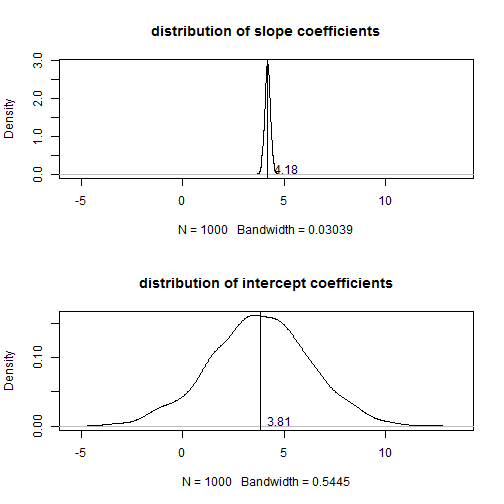par(mfrow = c(1, 1))


Now we see just how uncertain our intercept estimate is compared to the slope estimate.

Finally, why don't we go ahead and plot all 1000 fitted lines over the original data along with our original fitted line and the traditional 95% confidence band we get using our original model.

plot(x, y, col = "red", pch = 19)
abline(mod)  # original fitted line
apply(coef(modSim), 2, abline, col = "gray", lty = 3)

# predicted responses from original model
modOrig <- predict(mod, interval = "confidence")